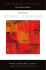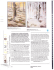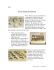# Mapping the Senses

## Transcription

Mapping the Senses
```,'.r:;;rni,*"
MapLi"g
,theUenSeS
Malcolm Liepke eharts the emotional
expressiveness of the human landscape
LIEPKE'S i-pr"rrive figurativework extendsbeyondthe clasMALCOLM
sicallyinspiredwork of his heroes-Whistler,Yel6.zquez,
Sargent,Schiele,and the
Japanese masters-to forge a strikingly modern vernacular. He explores the emotional
fabric of our lives and charts the intimate moments when we feel unobserved. When
viewing his paintings, we experience a sharp flash of recognition. "Malcolm's work has
always been about the emotional connection he is able to create between his paintings
and his viewers," says Steve Diamant of Arcadia Fine Arts in New York, which has represented Liepke for close to 20 years. "His juicy brushwork makes even the paintings'
surfaces sensuous."
Liepke, who loves early modern architectural design and feels it has influenced his
art; lives and works in a Prairie School home in suburban Minneapolis. The Prairie
School style, considered the first uniquely American residential architectural style,
evolved from the handcrafted, meticulous design and construction prevalent during the
early years of the 20th century and is closely identified with Frank Lloyd Wright. It features flowing interior spaces and clean lines, often with well-defined vertical elements
that mimicJapanese shoji screens. Liepke's home is the perfect setting for his studio and
artwork because these very qualities are often echoed in his paintings: It is not unusual
for the artist to juxtapose flowing human figures alongside or in front of geometric patterns and shapes, and to employ vertical gestural components for emphasis and rhythm.
Liepke's studio is not a showplace for fans to tour. It's a private, working studio where
only family members are allowed. It's a place where ideas are
enacted on canvas, where the artist sorts mental images like
apuzzle master, trying a piece here and there, turning it this
way and that, until, finally, it pops into place as his larger vision comes into focus. Liepke describes his
studio as very eclectic and personal. "It's
messy, sloppy even, with paintings all over
"Hisjuicybrushwork
makeseventhe paintings'
surfaces
sensuous."
DOSgtlR
REPRESENTATION
New York,NY;AtbemarleGallery,London,England.
uPcoMtNG sHows
Sotoshow,TelturideGalteryof FineArt, Februaryl-March 20
Soloshow,AlbemarleGallery,mid-May.
69 WWW.SOUTHWESTART.COM
FEBRUARY
2O1O // u/W\v.SOUTHWESTART.COM
63
h,:s
* i-.f',qr1fftr
:f .tIr;"
ard*
rr'a
f
rdt"h
: q
'.{@t.d,-N\$r
r
F:
\
|\
e
d ll,f-*t,*.*#
DREAMING.
l l r c l ] o o r r t n c l s t e e l < c ccl v c r v s h c r c . " h c s l r l s . " , \ t o n c c n c l o l t h e
l t r o n r i s i r s t ( l n c l i l c P l u c c u i l l r r r c l r r v c n p o r ll r n c l r r b i g - s el c e n l \ ' .
OIL.
18 X 24
o n l v r r b r r r t tl l l p c f e c n t o l i t . l h c r c ' s a l t r t o l c r r n f c n r l : r l irot ln . ( ) n t c
l ' r ' c u o r l i c c l o t t l n r ) e ( l n t ' c p t , t h c P l r i n t i n u g o c s l : r s t . l l r r r t l l : r s l ro l
r r n c l: r t t l r c o t l r c r - c n c li s n r t ' h u n r o r r g o u sl i h r e r r ' . " l h c r c a l c s h el v c s
r t n c ls h c l r c s o l : r r t h t r L r l < sI l.e h : L su n r : r s s c cclv c f ) ' t h i n g h c t l r n l i r r c l
c \ c c r r t i ( ) 1 rc r r n r c sc l L l i c ll<r " '
: t b o t L t1l t c l t l t i s t s ] r c l , r v c s .i l t t i n t c s s p c n c li n u v e l t r s h L r n ti r r q c l t r u n
: r r l r r c h t r r r kt o r r c l cflo h i s e o l l c e t i o r r .
s h o t r t s r t l L r t t r l p h L r t o s . " l r a r c l r ' h e v c e n r r ' r c l c sl i t l ( ) r r l l c n g t h v
1 - r 0 s c , l"t c c x l r l l t i n s . " ' l - h i r t c t i l r L i r u s c i l s e c l t c t ( ) q t ' r s t l t l c . t L r l O s c
I h c t - c n r r t i n i n gw e l l s t r l t h c s t u c li ( ) r t r - ce ( ) \ ' c l c c ul i t h p e i n t i n u s
l r n c l p t ' i n t s , l t n c l o n c i l r c i t s c r v c s l r s l r n " i c l c r s p r r e c " u h c r c h e -1 - r i n s
L r I s l i c t eh c s , P l r o t o s , i l n c i s r ' i l t l c f c a l n L r t c s l r b o L r t p o s s i b l c l L r t L l r c
p : r i n fi r . r { sl.l c r r o r l < se n t i f c l v i n r r r t i l i c i r rl li g h L ,a n c le l l o l r h e s i n c l ( ) \ \ ' si n t h c s t L r c l i or r r c t : r l r c c L
l r p ." \ \ ' h c n I l i g h t n r v l i g L r r e s ,I c r c i l t c n r \ ' ( ) \ \n Y i s i ( ) r (. )r l f c i l l i t \ ' , " s . t V St h c i r r l i s f. " l h c r c ' s n O t l t l O t L r l '
c l c c I s h r t c l o u( ) r n L l . u r e c\.l r ' I t o c c s * i s n r r r s t l vr r b o i L t h c s l r L r t g l c
t r l q c 1t i n g o L r tu l r r r t| \ \ ' . 1 1 1
r 1o s l l \ - t h c
64
WWW.SOUTHWES
'AIIT.COM
l r r ' to l P : r i n ti n g i s p r t r b . r b l t
FEBR,UARY
2O1O
l - i c p l i e u s c s b o t h p r o l c s s i L r n a l r n c l l t ( ) l t p r ( ) l r s s i r ) n r rnl r o c l c l sr r n c l
i t s l r c s h n c s s r l n r l i n m c c l i r c r ' . " I l c l i r s t h l o c l < s i n r r n i c l c r rr r n c l
t l r c r r r c s o ] r ' c sl t n V e o r l p o s i t i t l n a l p r o b l c n r s a s h c u o c s . I : n t p l o v i r r u
his Lru'nlrighlr'pcrsonill scnsc ol color, thc ltrtisl LrcelrsiLlnlrllv
t l r r o u s i n r r c l a s h L r l 't l r c u n c x p r c c t c c l , r r v i s u r r l s u r p r i s c s i t h i n r r
c l o n r i n . r n t p e l c t t c o l ' g r c c r r s , g r i t v s , l l r c l b l l c ] < s . I l c u o r l < s\ \ ' c t i n t i )
r r c t . r r s i n s u l o u c l c c bl r u s h t r r : r c h i c v ct h c l L r s h ,p a i n t c l l v l t r o l it h r r t
i s h i s s i q n i l t L u ' c \. \ ' h e n p r c p e r i n g l o r : r s l r o r r ' .h c o l t c n I r i r sl ( l t i r
l l l e l t n v l t s c s i n p r i r ec s s i l t ( ) n r : c .
nuBY LrPS, OtL,24 X 24.
LIEPKE
GREW
UP in Minnesota.
His parents
were
designers who created seasonal d€cor for shopping malls all over
the country. As a youngster, Liepke excelled in two rhingssports and art. He Ionged to become a professional athlete, but
as he grew older he realized it was not to be. Art came easily, and
his teachers encouraged him. 'Art chose me," he says. He had
considered becoming an architect, but after seeing a brochure
he decided to head west to study art instead. However, it wasn't
qulte the experience he had envisioned. It was an era when art
education had turned from classical training to conceptual art,
and Liepke had always been drawn to figurative work. "But they
made me focus and gave me a craft as an illustrator," he says.
He hung around L.A. for awhile, working as a commercial art-
ist, even taklng a turn creating posters for movies and illustrations
for such well-known TV shows as RichMan, PoorMan,starring Nick
Nolte. But the West Coast scenejust wasn't for him. New York beck
oned. Once there, "Things just exploded!" he says. "Everyone'son
their own road to self-discovery. It's different for each individual.
When I moved to New York, I started going to museums and learning from all the great artists. I learned color, composition, and technique. I realized their work was my kind of work. They were my
heroes, so I became their student. I came to love the city. In fact, we
kept an apartment there until just a few years ago."
In New York, Liepke was a highly sought-after illustrator. His
work appeared on the covers of Trme,Newsweek,Forbes,Fortune, and
Sportslllustrated,
among others. He did well, but eventually he tired
of the compromises required by the corporate world. "Great art
E.EBR'UARY zOtO // u/WW.SOUTHWESTART.COM
05
,
,r,l
/,t
f
R-irr;"."-.,{#
f :
*.
I
I
i
'.1
I
I
r*id-
STUDY
IN MAUVES,
i s a b o u t o r . r cp er s o r . r 'rs" i s i o n , "h e c x p l l i n s . l l c u , r r n t c ctli r l ' o c r t so n
h i s o n ' r . ri n t c r p r c t a t i o r . o
r s1 ' at l r e m e. S o i r r t h c e a r l y ' 9 { . ) sh, c l c l t i l l L r s t r r t i r rbr rc h i n d a n d t u r n c d t o l i r - r er r r t l r - r l l t i m e .
F - r r gr et O t r y r l e w p r o c es s c s ,h c s t L r d i c dl i t h o g r e p h y .I l c g a i n c d
l c c c s s t o t u ' o l r r . r t i c l uM
e: r r i n o n i \ / o i r i n p r c s s c s - t h c s a r r c t y p e
-l-rrulousc
I - a u t r c cu s e d - r n d b e g r n p m d u c i n g i r b o r - i n t e n s i v c ,
h r r n d - d r r r u ' np l a t e s o f el e g a n t , g r a c el u l i n r a s c s .l l L r tc . l c h i n r a g c
f o o l <n r t - r n t htso p r o d u c e . , r n dh e d i d n ' t r e r r l l yc n . j o yt h c p r ( ) c c s s .
I t n ' r r s t i r . r . rtco c l e v o t eh i m s e l l - t o h i s t r u e p r s s i o n - l ' i g r - r r a t i v e
1--rrrinting.
I t r d r y L i e p k e e x h i b i r s i n t e r n a t i o n a l l ya r - r chl r s r c c c i v c d m a n y
au'arclsirrd rccolades that rellect lris gron,ing stiltLrsrrsa signific r r n tA r n er i c r n a r t i s t . l l i s p a i n t i n g sr r e i n t h e p c r m a n e n t c o l l e c t i o n s o f t h e N a t i o r - r aAl c a d e m yo f D e s i g n ,t l - r eR r o o l < i y nM u s e u r n ,
a n d t h e S m i t h s o n i r n ,a n d i n t h e p r i v a t ec o l l e c t i o r - rosl s u c h c e l e b r i t i e s a s l l a r b r aS t r ei s a n d a n d J a r l e sB r o l i r r ,l ) o n n : rK : r r a n ,l l r o o k e
66
aA.
OIL, 14 X 16.
WWW.SOUTHWESTART.COM
// FEBRUARY
2O1O
> h i c l t l t ,: t r r r l ) i r l n t l , r r r r ' .
()vel tlrc past clecadc,l-iepke's u'orl< I-ursbcconrc richcr, nrorc
serrsual,a nd rnorc evoc:ttive. LJnt i I qu ite receIrtly nrostof h is cotrpositions leatr,rrcdonc or t\\,o central figr-rres(oftcr-rernphasizcd
i r - rs t l r t l i n g c ( ) n t r i l s ta g a i n s ta b r c l < g r o u n do f g eo m c t r i c p r l t t c r n s
encl shapes)or small groups of people errgagedin conversrttit'rt.t
i n a b u s y b a r s c e n c .P a r t i c r , r l a rcl yo m p e l l i n ga r e h i s p a i n t i n g s
o f l o n e f e r n a l ef i g r - r r e s - a l l s o l ' t ,c u r v i n g l i n e s u ' i t h g l o v l i n g , l r - r r.r.rir-rous
flesh irnd lr-rshlips-caught unirr'r'arein rlr intinrite m\)ment and rendered in r l-lr-rrry
o[ articr-rlated,tlashy brushutrl<.
" I l o v e d r a m a t i c g es t u r e , " s a y sL i e p k e ," a n d I c o n s c i o u s l ys c : r r c h
o r - r tc o n r p o s i t i o n a d
l e v i c e st h a t u ' i l l h e i g h t e n a n d s t r c n g t h c n r
pai r-rting's cmc-rtit'lnrl
impact."
I [ e c o ] l e c t s . . l a p a n e sper i n t s a n d a n t i q u e sa n d i s r r h u g e f r r n , r l . J r r p a n e saer t i s t s s u c h a s H o k u s a i , U t a m r r o , a n d S h a r a k u .H e i s
drau'n to tlreir r-rnderstatedstyle and satnrirted color, the care-
cLosEUP,
OrL, 18 X 28.
fully thought-out use of black, and rhe inherent sense of design
that is so characteristic of their work. He has found similar ways
to approach his own paintings, accenruaring rheir highly emotional aspects through the angles of his subjects' arms, bodies,
and expressive hands. He maps the geometry of this human
landscapethrough repetition of gesture, angle, and line, and underscores it through careful attention to negative space. If you
squint, each painting appears a well-balanced, coherent design
irrespective of figurative content, making the piece emotionall%
intellectually, and visually satisfying.
In his most recent images, Liepke zeroes in on that most telling component of the human figure-the face. In these pieces he
feels less need for a narrative setting and employs looser, wilder
brushwork to further animate the subject's emotional expressiveness. Considering such paintings as DREAMING, OVER HER
SHOULDER,or RUBYLIPS,it's easy to see he has closed in on the
crux of his style and is painting it boldly with a masrer's experienced hand. These faces are voluptuous and juicy and totally
arresting. As Steve Diamant says, "Liepke is distilling down to
the core essenceof what attracts people to his work. He,s putting
a magnifying glass on this essenceby going right to the face."
Early cartographers made maps that dreamed of an unknown
physical world, while later geographers satisfied their dreams
with carefully detailed drawings of every hill and warerway.
Malcolm Liepke mines his world for emotional content, sweeping brush across canvas to reveal private thoughts and imaginings, and, in the process, reveals himself. -:.
Ilosornrrrv
Clar"stons, cclitor
oJ' the ilurarc.l-n,irrning
u'ritin13:r book alrout Mcxi<:o City:rrtist;
r.ml)olrr'\:
of Dicgo Rivcra
Annctto
and F'ridlr Ka.hlo-
u'ebzinc
G:
F'l'lAS'I', is
N:rncarrrlu',:u
con-
OVER HER SHOULDER, OIL, 11 X 9.
FEBR'UAn,Y 9O1O // u/u/v/.SOUTHWESTART.COM
6?
```

### Glass Houses 24: Geoff Hippenstiel### After Joris Hoefnagel and Georg Bocskay Queen of Spain fritillary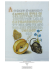### Paintings of Orient by Hovsep Pushman### Jan Schall, Wilbur Niewald, 10/Charlotte Street Foundation, 2007### Untitled - Clifton Diocese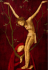### Untitled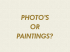### Pissarro was born in the caribbean and moved to paris when he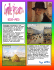### TOLLAND COUNTY ART ASSOCIATION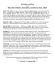### Oyfn veg shteyt a boym, Sung by Chava Alberstein ILEX BELLER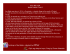### Extended Abstract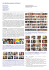### March 2010 - Virginia Watercolor Society### Where Women Create - Geri Lindsey`s website. A native of the### review of exhibitions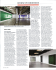### From The Air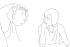### the Studio Tour Brochure/Map### "bst 2016" brochure pdf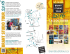### Studio Art Tour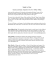### Norval Morriseau Info and Project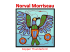### TAI-SHAN SCHIERENBERG LOS PADRES LYNN DENNISON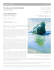### michael quadland - Behnke • Doherty Gallery